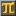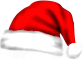#SCIENTIFIC CALCULATORThis calculation: Edit - Share - Print -
Save

• Press Ctrl-d to save this calculation as a bookmark.

- Reset

 Ways of re-using calculations: 180 / 3 =   60.0  A: Using ans sin(ans)**2 + cos(ans)**2 =   1.0  B: Clicking on equation number sin()**2 + cos()**2 =   1.0  C: Clicking on left side of equation  sin(180 / 3)**2 + cos(180 / 3)**2 =   1.0  D: Clicking on right side of equation  sin(60)**2 + cos(60)**2 =   1.0  These methods are not equal... int(rand * 1000) =   492.0  ... try methods A through D on equation .
CalculatorPi.comⒸ Troymius LLC